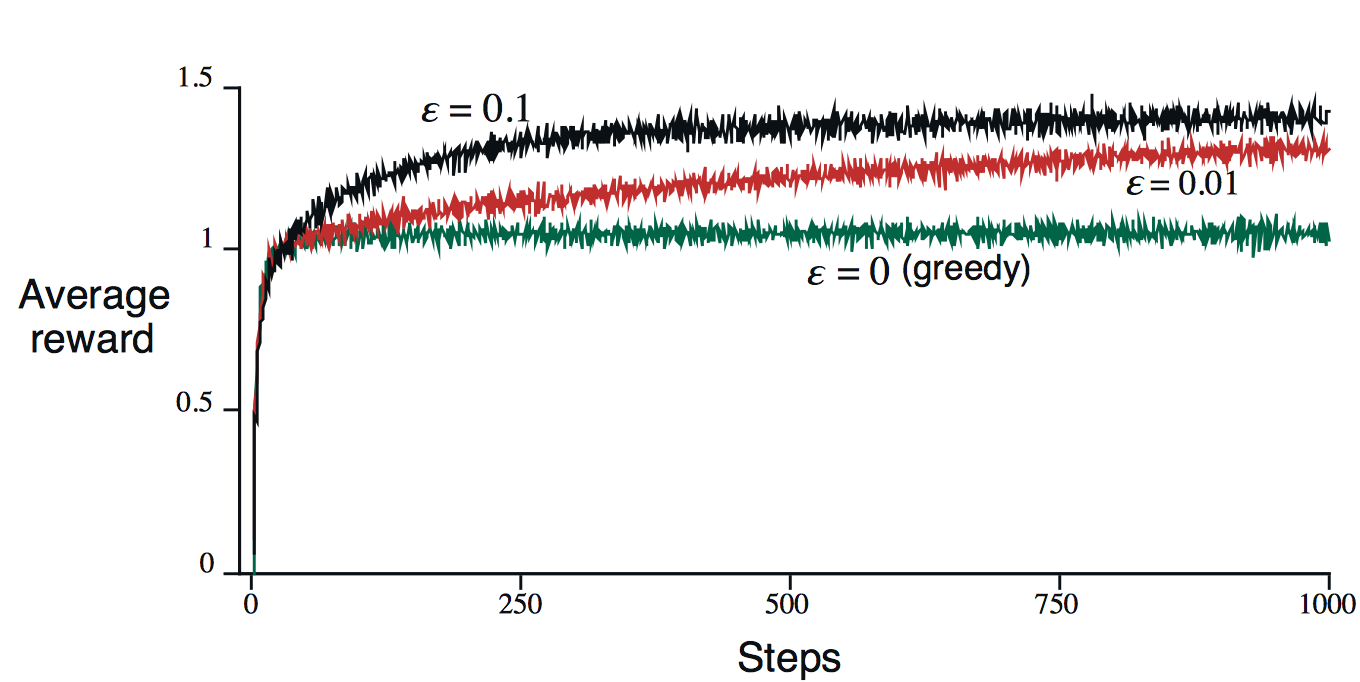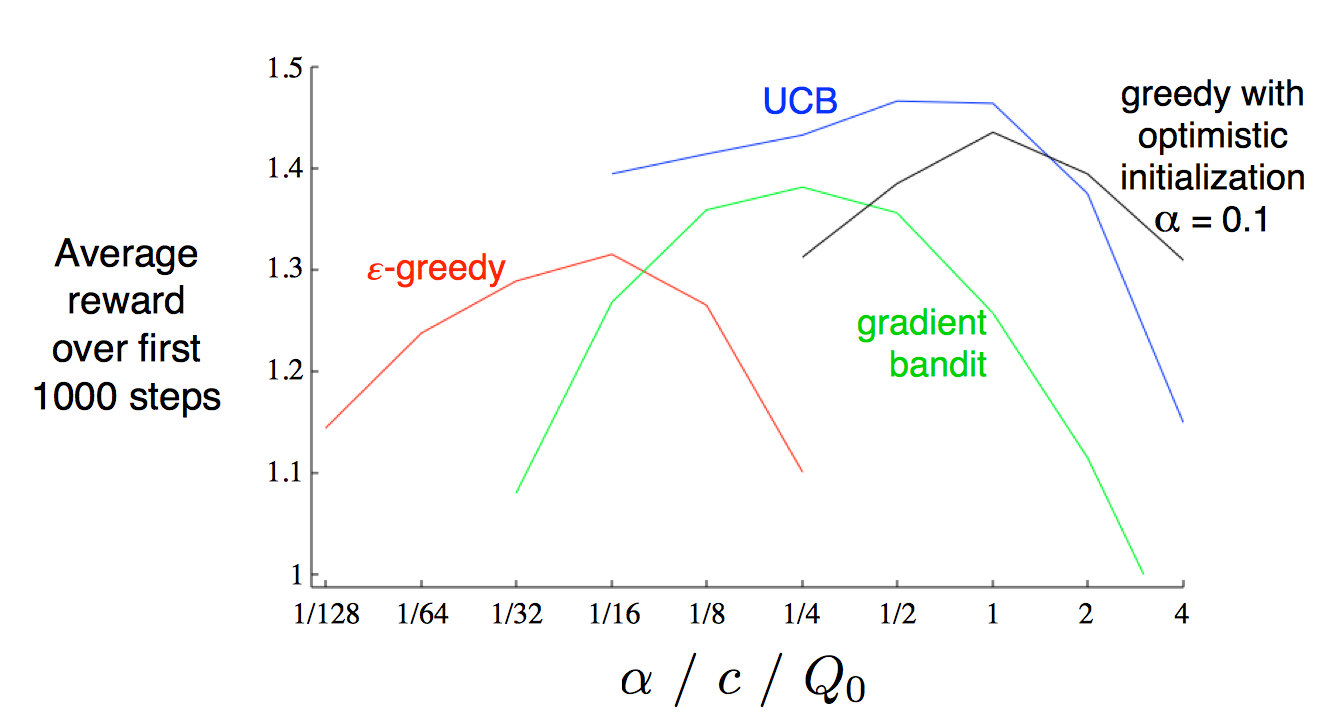# 目前给定的三个方向

• 流量识别(traffic classification)：使用不同的模型，根据在流量中观测到的不同特征以及自己的目标，进行分类。目前针对该问题使用的主流算法有：K-means, AutoClass, SVM(支持向量机)等。

根据目标不同，分类结果随之改变。如可以按照应用类别将流量分类为(HTTP, FTP, WWW, DNS, P2P)；按具体应用将流量分类为(Skype, YouTuBe, Netflix)。

通常，网络流量分类方法可以分为四大类，利用端口号、分组有效载荷、主机行为或流特征进行分类。

• 路径规划(route planning)：为分组的传输寻找到一条适合的路线。根据运营政策以及自己的目标不同，评判标准也不一致：最小化代价、最大化链路利用率、服务质量(Qos)的保证等。

路径规划在ML领域是一个具有挑战性的难题，因为需要应对动态变化的复杂拓扑网络、学习选定的路径与服务质量之间的关系、以及预测路径规划带来的结果。目前，强化学习(RL)主导了该领域的研究。

• 资源分配(resource allocation)：通过管理资源的分配以最大化长期目标，如收益或资源的最大利用率。这里的资源包括CPU、内存、磁盘、交换机、路由器、带宽、AP、广播频道及其频率。

资源分配的主要难题是在面对不可预测性的情况下调整资源以实现长期利益

# 基本概念

RL首先是非监督学习，这是因为评估每个行为时使用的是估计(evaluation)，我们只大概清楚采用某个行为的好坏程度，并且不确定这是最好行为还是最坏行为。与之相对，监督学习得到的则是指导性回馈(instrcutive feedback)，我们知道每一步的正确解，并且根据该结果修正参数。

## MDP

MDP是DTP(decision-theoretic planning)的一种非常直观并且重要的表现形式。在这个形式下，环境(environment)可以表现为状态以及行为的集合。包含了状态、行为、状态转移、奖励函数。将上述的四个因素结合在一起，便得到了马尔科夫过程，表示为一个元组$<S, A, T, R>$。

• states

状态表示描述当前形势的所有必要信息。表示为
$S = {s^1, …,s^N}$

其中$N$表示状态的总数。

• actions

行为用于控制系统状态。可以表示为${a^1, …,a^K }$。其中$K$表示行为的总数。使用$A(s)$来表示可以作用于状态$s$的行为集合。在某些系统中，不是所有状态都可以执行所有行为。

• transition function

状态转移函数定义：$T:S\times A \times S \rightarrow [ 0, 1]$

表示从状态$s$经过行为$a$转移到状态$s’$的概率。规定：

• $1\ge T(s, a, s’) \ge 0$
• $\sum_{s’\in S} T(s, a, s’) = 1$

系统被称为Markovian的，当且仅当系统的状态转移与以前无关，而只与当前的状态以及当前的行为有关。这个在动态规划中可以得到十分形象具体的解释。同时存在着k-Markov的系统，表示下一状态仅与前k个状态相关。

• reward function

定义：$R:S \times A \rightarrow \mathbb{R} \text{ or } R:S\times A\times S \rightarrow \mathbb{R}$

表示在某个状态执行某种行为获得的奖励，或者在一个状态通过某种行为到达另一种特定状态带来的奖励。

## Optimality Criteria

• $\displaystyle E \left[ \sum_{t=0}^h r_t \right]$

该目标函数从当前状态出发，考虑了接下来的$h$步决策，可以认为是考虑了局部最优的情况。属于贪心。

• $\displaystyle E\left[ \sum_{t=0}^{\infty}\gamma ^t r_t\right]$

该目标函数从当前状态出发，考虑了之后的所有可能情况，但是其中包含了$\gamma^t$项，表示随着步数的增加，赋予的权值越来越小($\gamma < 1$)。因此该目标函数虽然考虑了全局的情况，但是近期的优先级高于未来的优先级。

• $\displaystyle \lim_{h\rightarrow\infty}E\left[ \frac{1}{h}\sum_{t=0}^h r_t\right]$

该目标函数从当前状态出发，同样考虑了将来的所有情况，并且对每一步的优先级都平等对待。

# 多臂赌博机问题

## 问题引入

• 假设一个用户对不同类别的内容感兴趣程度不同，那么我们的推荐系统初次见到这个用户时，怎么快速地知道他对每类内容的感兴趣程度？这就是推荐系统的冷启动。
• 假设我们有若干广告库存，怎么知道该给每个用户展示哪个广告，从而获得最大的点击收益？是每次都挑效果最好那个么？那么新广告如何才有出头之日？
• 我们的算法工程师又想出了新的模型，有没有比A/B test更快的方法知道它和旧模型相比谁更靠谱？

## Action-Value Method

$$Q_t(a) = \frac{R_1 + R_2 + \dots + R_{N_t(a)}}{N_t(a)}$$

\begin{equation}\begin{aligned}Q_t(A_t^ )& = \max_aQ_t(a) \ A_t&=\mathop{\arg\max}_a Q_t(a)\end{aligned}\end{equation}$\epsilon -greedy$与$greedy$算法也有不同的使用场景。如果每个“老虎机”没有偏差而给出的都是固定收益，那么肯定只需要使用$greedy$算法对每个“老虎机”操作一遍即可得到最优行为。如果偏差很大，那么$\epsilon-greedy$算法可以更快地找到最优行为。

## Incremental Implementation

\begin{equation}\begin{aligned}Q_{k+1}&=\frac{1}{k}\sum_{i=1}^kR_i \ &=\frac{1}{k} \Bigg(R_k + \sum_{i=1}^{k-1}R_i \Bigg) \ &= \frac{1}{k}\Big(R_k + (k - 1)Q_k + Q_k - Q_k\Big) \ &= \frac{1}{k}\Big( R_k + kQ_k - Q_k\Big) \ &=Q_k + \frac{1}{k}\Big[R_k - Q_k \Big]\end{aligned}\end{equation}

## Upgrade: Nonstationary Problem

$$Q_{k+1} = Q_k + \alpha \Big[R_k - Q_k \Big]$$

$$Q_{k+1} = (1-\alpha)Q_k + \alpha R_k$$

## 对比采样平均与指数加权平均

$$\sum_{k=1}^\infty\alpha_k(a) = \infty \ \ \ \ \text{and} \ \ \ \ \sum_{k=1}^\infty\alpha_k^2(a) < \infty$$

## Upper-Confidence-Bound Algorithm

$$A_t = \mathop{\arg\max}_a\Bigg[Q_t(a) + c\sqrt{\frac{\ln t}{N_t(a)}} \ \Bigg]$$

\begin{equation} \begin{aligned} P(\mu \ge \hat{\mu} + \epsilon) &= P(\frac{S_n}{\sqrt n}\le -\epsilon \sqrt n) \ &= P(\frac{S_n}{\sqrt n} \ge \epsilon \sqrt n) \end{aligned} \end{equation}

$$P(\mu \ge \hat{\mu} + \epsilon) \le \exp(\frac{-n\epsilon^2}{2})$$

$$P(\mu \ge \hat{\mu} + \sqrt{\frac{2}{n} \ln \frac{1}{\delta}}) \le \sigma$$

$$\Pr{A_t = a} = \frac{e^{H_t(a)}}{\sum_{b=1}^{n}e^{H_t(b)}} = \pi_t(a) \tag{1}$$

\begin{equation} \begin{aligned} H_{t+1}(A_t) &= H_t(A_t) + \alpha (R_t - \overline{R}_t)(1-\pi_t(A_t)) \ H_{t+1}(a) &=H_t(a) - \alpha (R_t - \overline{R}_t)\pi_t(a), & \forall a \ne A_t \end{aligned} \end{equation} \tag{2}

$$H_{t+1}(a) = H_t(a) + \alpha\frac{\partial\mathbb{E}[R_t]}{\partial H_t(a)} \tag{3}$$

$$\mathbb{E}[R_t] = \sum_b \pi_t(b)q(b)$$

\begin{equation} \begin{aligned} \frac{\partial\mathbb{E}[R_t]}{\partial H_t(a)} &= \frac{\partial}{\partial H_t(a)}\Bigg[\sum_b\pi_t (b)q(b) \Bigg]\ &= \sum_bq(b)\frac{\partial\pi_t(b)}{\partial H_t(a)} \end{aligned} \end{equation} \tag{4}

\begin{equation} \begin{aligned} \frac{\partial \pi_t(b)}{\partial H_t(a)} &= \frac{\partial}{\partial H_t(a)}\pi_t(b) \ &=\frac{\partial}{\partial H_t(a)}\Bigg[\frac{e^{H_t(b)}}{\sum_{c=1}^n e^{H_t(c)}} \Bigg] \ &= \frac{\frac{\partial e^{H_t(b)}}{\partial H_t(a)}\sum_{c=1}^n e^{H_t(c)}- e^{H_t(b)}\frac{\partial \sum_{c=1}^n H_t(c)}{\partial H_t(a)}}{(\sum_{c=1}^ne^{H_t(c)})^2} \ &= \frac{\mathbb{I}_{a=b} e^{H_t(a)} \sum_{c=1}^n e^{H_t(c)} - e^{H_t(b)}e^{H_t(a)}}{(\sum_{c=1}^ne^{H_t(c)})^2} \ &= \frac{\mathbb{I}_{a=b}e^{H_t(b)}}{\sum_{c=1}^ne^{H_t(c)}} - \frac{e^{H_t(b)}e^{H_t(a)}}{(\sum_{c=1}^ne^{H_t(c)})^2} \ &= \mathbb{I}_{a=b}\pi_t(b) - \pi_t(b) \pi_t(a) \ &= \pi_t(b) (\mathbb{I}_{a=b}-\pi_t(a)) \end{aligned} \end{equation}

$$\sum_b \frac{\partial\pi_t(b)}{\partial H_t(a)} = 0$$

\begin{equation} \begin{aligned} \frac{\partial\mathbb{E}[R_t]}{\partial H_t(a)} &= \sum_bq(b)\frac{\partial\pi_t(b)}{\partial H_t(a)} \ &= \sum_b (q(b) - X_t) \frac{\partial\pi_t(b)}{\partial H_t(a)} \ &= \sum_b \pi_t(b)(q(b) - X_t) \frac{\partial\pi_t(b)}{\partial H_t(a)} / \pi_t(b) \end{aligned} \end{equation}

\begin{equation} \begin{aligned} \frac{\partial\mathbb{E}[R_t]}{\partial H_t(A_t)} &= \mathbb{E}\Bigg[ (q(A_t) - X_t)\frac{\partial\pi_t(A_t)}{\partial H_t(a)} / \pi_t(A_t)\Bigg] \ &= \mathbb{E}\Bigg[(R_t - \overline{R}_t)\frac{\partial\pi_t(A_t)}{\partial H_t(a)} / \pi_t(A_t) \Bigg] \ &= \mathbb{E}\big[(R_t - \overline{R}_t)\pi_t(A_t)(\mathbb{I}_{a= A_t} - \pi_t(a)) /\pi_t(A_t)\big] \ &= \mathbb{E}\big[(R_t - \overline{R}t)(\mathbb{I}{a=A_t} - \pi_t(a)) \big] \end{aligned} \end{equation}

\begin{equation} \begin{aligned} H {t+1}(a) &= H_t(a) + \alpha (R_t - \overline{R}t)(\mathbb{I}{a = A_t} - \pi_t(a)), & \forall a \end{aligned} \end{equation}

## Summary0%